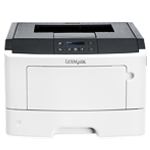Lexmark MS410

UseTo

Plain Weight

• Light
• Normal
• Heavy

Specify the relative weight of the plain paper loaded.

Note:  Normal is the factory default setting.

Card Stock Weight

• Light
• Normal
• Heavy

Specify the relative weight of the card stock loaded.

Note:  Normal is the factory default setting.

Transparency Weight

• Light
• Normal
• Heavy

Specify the relative weight of the transparencies loaded.

Note:  Normal is the factory default setting.

Recycled Weight

• Light
• Normal
• Heavy

Specify the relative weight of the recycled paper loaded.

Note:  Normal is the factory default setting.

Labels Weight

• Light
• Normal
• Heavy

Specify the relative weight of the labels loaded.

Note:  Normal is the factory default setting.

Bond Weight

• Light
• Normal
• Heavy

Specify the relative weight of the bond paper loaded.

Note:  Normal is the factory default setting.

Envelope Weight

• Light
• Normal
• Heavy

Specify the relative weight of the envelope loaded.

Note:  Normal is the factory default setting.

Rough Envelope Weight

• Light
• Normal
• Heavy

Specify the relative weight of the rough envelope loaded.

Note:  Normal is the factory default setting.

• Light
• Normal
• Heavy

Note:  Normal is the factory default setting.

Preprinted Weight

• Light
• Normal
• Heavy

Specify the relative weight of the preprinted paper loaded.

Note:  Normal is the factory default setting.

Colored Weight

• Light
• Normal
• Heavy

Specify the relative weight of the colored paper loaded.

Note:  Normal is the factory default setting.

Light Weight

• Light

Specify that the weight of the paper loaded is light.

Heavy Weight

• Heavy

Specify that the weight of the paper loaded is heavy.

Rough/Cotton Weight

• Light
• Normal
• Heavy

Specify the relative weight of the cotton or rough paper loaded.

Note:  Heavy is the factory default setting.

Custom [x] Weight

• Light
• Normal
• Heavy

Specify the relative weight of the custom paper loaded.

Notes:

• Normal is the factory default setting.
• The options appear only when the custom type is supported.